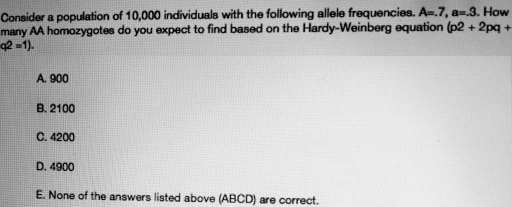# Problem: Consider a population of 10,000 individuals with the following allele frequencies: A = 0.7, a =0.3. How many AA homozygotes do you expect to find based on the Hardy-Weinberg equation (p2 + 2pq + q2 = 1)A. 900B. 2100C. 4200D. 4900E. None of the answers listed above are correct

###### FREE Expert Solution

Following Hardy-Weinberg equilibrium,the relative frequencies of each genotype can be estimated from the relative frequencies of the allele. The equation follows p2 + 2pq + q2 = 1, where p and q are the relative frequencies of the dominant and recessive allele, respectively.###### Problem Details

Consider a population of 10,000 individuals with the following allele frequencies: A = 0.7, a =0.3. How many AA homozygotes do you expect to find based on the Hardy-Weinberg equation (p2 + 2pq + q2 = 1)

A. 900

B. 2100

C. 4200

D. 4900

E. None of the answers listed above are correct